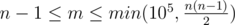CF160D Edges in MST

• 50通过
• 151提交
• 题目来源
• 评测方式 RemoteJudge
• 标签 并查集 生成树 线段树 连通块
• 难度 省选/NOI-
• 时空限制 2000ms / 256MB
• 提示：收藏到任务计划后，可在首页查看。

题意翻译

题目大意:

给一个带权的无向图,保证没有自环和重边. 由于最小生成树不唯一,因此你需要确定每一条边是以下三种情况哪一个 1.一定在所有MST上 2.可能在某个MST上 3.一定不可能在任何MST上 输入

第一行给出n,m表示点数和边数. 数据范围见原题面 之后m行,每行给出ai,bi,wi 表示一个边的两个端点和边的权值.保证没有自环与重边. 输出

你需要输出m行,每行依次表示输入的第几条边,如果是情况1,输出 "any"(不包含引号);如果是情况2,输出 "at least one"(不包含引号);如果是情况3,输出 "none"(不包含引号).

题目描述

You are given a connected weighted undirected graph without any loops and multiple edges.

Let us remind you that a graph's spanning tree is defined as an acyclic connected subgraph of the given graph that includes all of the graph's vertexes. The weight of a tree is defined as the sum of weights of the edges that the given tree contains. The minimum spanning tree (MST) of a graph is defined as the graph's spanning tree having the minimum possible weight. For any connected graph obviously exists the minimum spanning tree, but in the general case, a graph's minimum spanning tree is not unique.

Your task is to determine the following for each edge of the given graph: whether it is either included in any MST, or included at least in one MST, or not included in any MST.

输入输出格式

输入格式：

The first line contains two integers $n$ and $m$ ( $2<=n<=10^{5}$ ,) — the number of the graph's vertexes and edges, correspondingly. Then follow $m$ lines, each of them contains three integers — the description of the graph's edges as " $a_{i}$ $b_{i}$ $w_{i}$ " ( $1<=a_{i},b_{i}<=n,1<=w_{i}<=10^{6},a_{i}≠b_{i}$ ), where $a_{i}$ and $b_{i}$ are the numbers of vertexes connected by the $i$ -th edge, $w_{i}$ is the edge's weight. It is guaranteed that the graph is connected and doesn't contain loops or multiple edges.

输出格式：

Print $m$ lines — the answers for all edges. If the $i$ -th edge is included in any MST, print "any"; if the $i$ -th edge is included at least in one MST, print "at least one"; if the $i$ -th edge isn't included in any MST, print "none". Print the answers for the edges in the order in which the edges are specified in the input.

输入输出样例

输入样例#1： 复制
4 5
1 2 101
1 3 100
2 3 2
2 4 2
3 4 1

输出样例#1： 复制
none
any
at least one
at least one
any

输入样例#2： 复制
3 3
1 2 1
2 3 1
1 3 2

输出样例#2： 复制
any
any
none

输入样例#3： 复制
3 3
1 2 1
2 3 1
1 3 1

输出样例#3： 复制
at least one
at least one
at least one


说明

In the second sample the MST is unique for the given graph: it contains two first edges.

In the third sample any two edges form the MST for the given graph. That means that each edge is included at least in one MST.

提示
标程仅供做题后或实在无思路时参考。
请自觉、自律地使用该功能并请对自己的学习负责。
如果发现恶意抄袭标程，将按照I类违反进行处理。